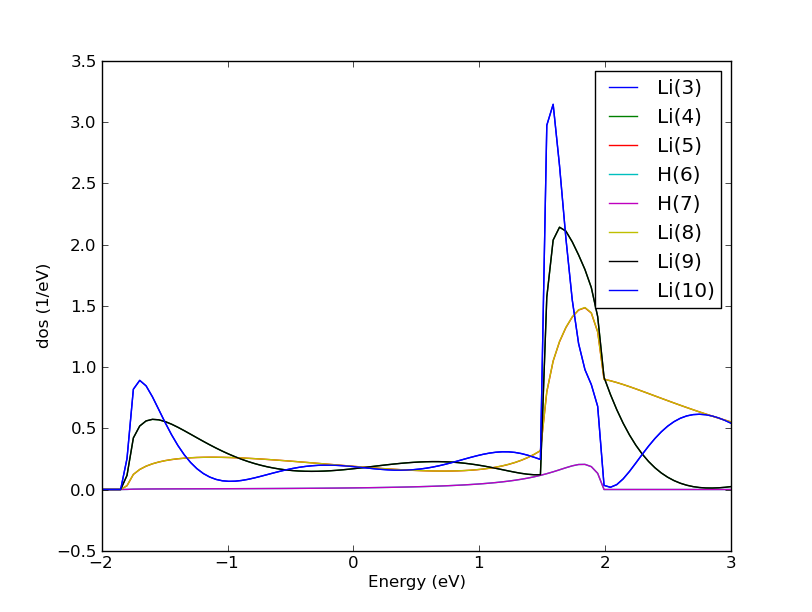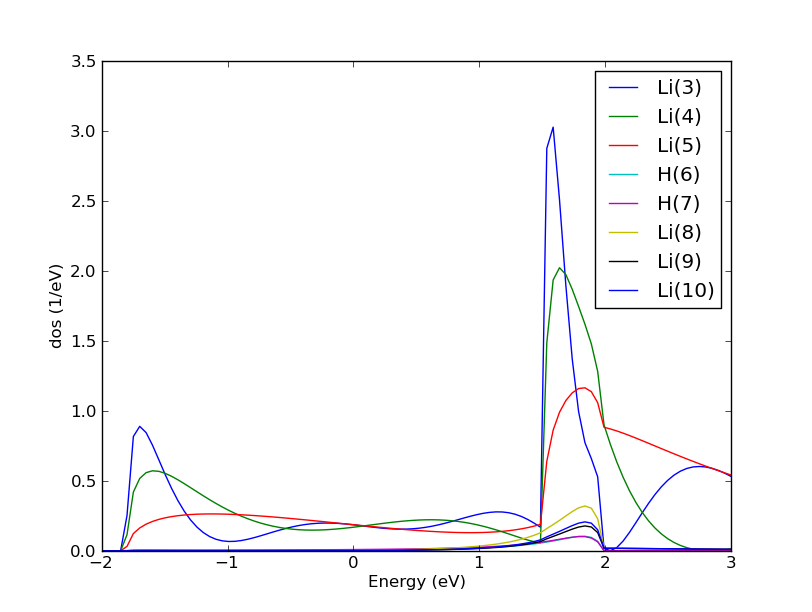# DeviceDensityOfStates¶

class DeviceDensityOfStates(configuration, energies=None, kpoints=None, contributions=None, self_energy_calculator=None, energy_zero_parameter=None, infinitesimal=None)

Constructor for the Device Density of States object.

Parameters: configuration (DeviceConfiguration) – The one or two-probe configuration with attached calculator for which the density of states. should be calculated. energies (PhysicalQuantity of type energy.) – The energies for which the density of states should be calculated. Default: numpy.linspace(-2., 2., 200)*eV kpoints (MonkhorstPackGrid | RegularKpointGrid) – The k-points for which the density of states should be calculated. Default: MonkhorstPackGrid(nx, ny), where nx, ny is the sampling used for the self consistent calculation. contributions (Left | Right | All) – The density contributions to include in the density of states. Default: All self_energy_calculator (DirectSelfEnergy | RecursionSelfEnergy | SparseRecursionSelfEnergy | KrylovSelfEnergy) – The self energy calculator to be used for the density of states. Default: RecursionSelfEnergy(storage_strategy=NoStorage()) energy_zero_parameter (AverageFermiLevel | AbsoluteEnergy.) – Specifies the choice for the energy zero. Default: AverageFermiLevel infinitesimal (PhysicalQuantity of type energy.) – Small energy, used to move the density of states calculation away from the real axis. This is only relevant for recursion-style self-energy calculators. Default: 1.0e-6*eV
bias()
Returns: The applied bias. PhysicalQuantity type voltage.
electrodeFermiLevels()
Returns: The Fermi levels of the left and right electrodes in absolute energies. PhysicalQuantity of type energy.
electrodeFermiTemperatures()
Returns: The Fermi temperature of the left and right electrodes used in this density of states. PhysicalQuantity of type temperature.
electrodeVoltages()
Returns: The left and right electrode_voltages used in this density of states spectrum. PhysicalQuantity of type voltage.
elements()
Returns: The elements in the configuration used for generating the density of states. list of PeriodicTableElement
energies()
Returns: The energies used in this density of states. PhysicalQuantity of type energy.
energyZero()
Returns: The energy zero used for the energy scale in this density of states. PhysicalQuantity of type energy.
evaluate(spin=None, projection_list=None)

Evaluate the density of states.

Parameters: spin (Spin) – The spin component. Default: Spin.Sum projection_list (ProjectionList) – ProjectionList with projections to include in the density of states. Default: ProjectionList(range(n_left, n_central-n_right)) The density of states for the given spin component. For the spin Spin.All, a list containing the density of states for Spin.Sum, Spin.X, Spin.Y and Spin.Z is returned. PhysicalQuantity | list(4) of PhysicalQuantity
infinitesimal()
Returns: The infinitesimal used for calculating the density of states. PhysicalQuantity of type energy.
metatext()
Returns: The metatext of the object or None if no metatext is present. str | unicode | None
nlprint(stream=None)

Print a string containing an ASCII table useful for plotting the AnalysisSpin object.

Parameters: stream (python stream) – The stream the table should be written to. Default: NLPrintLogger()
numberOfElectrodeAtoms()
Returns: The number of atoms in each electrode in the configuration used for generating the density of states. tuple of int.
setMetatext(metatext)

Set a given metatext string on the object.

Parameters: metatext (str | unicode | None) – The metatext string that should be set. A value of “None” can be given to remove the current metatext.
spins()
Returns: The spins used in this density of states. tuple of spin component flags.

## Usage Examples¶

Calculate the DeviceDensityOfStates (DDOS) of a Li-H2-Li system:

# setup Huckel calculation for Li-H2-Li device

electrode_lattice = UnitCell([8., 0., 0.]*Angstrom,
[0., 8., 0.]*Angstrom,
[0., 0., 8.7]*Angstrom)

electrode_elements = [Lithium, Lithium, Lithium]
electrode_coordinates = [ [ 4.,  4., 1.45+i*2.9] for i in range(3)]*Angstrom

electrode = BulkConfiguration(
bravais_lattice=electrode_lattice,
elements=electrode_elements,
cartesian_coordinates=electrode_coordinates
)

lattice = UnitCell([8., 0., 0.]*Angstrom,
[0., 8., 0.]*Angstrom,
[0., 0., 37.376]*Angstrom)

elements = [Lithium,]*6 +[Hydrogen,]*2+[Lithium,]*6

coordinates = [ [ 4.,  4., 1.45+i*2.9] for i in range(6)] + \
[ [ 4.,  4., 18.286+i*0.8] for i in range(2)] + \
[ [ 4.,  4., 21.426+i*2.9] for i in range(6)]

central_region = BulkConfiguration(
bravais_lattice=lattice,
elements=elements,
cartesian_coordinates=coordinates*Angstrom
)

device_configuration = DeviceConfiguration(
central_region,
[electrode, electrode]
)

calculator = DeviceHuckelCalculator(
iteration_control_parameters = NonSelfconsistent,
)

device_configuration.setCalculator(calculator)

device_configuration.update()
nlsave('device.nc', device_configuration)

#setup energies, and calculate DOS
energies = numpy.linspace(-2,3,100) * eV
dos = DeviceDensityOfStates(device_configuration, energies=energies)
nlsave('device.nc', dos)


device.py

Plot the projected density of states (PDOS) for the non-surface atoms of the central region:

# read the dos
conf = nlread('device.nc', DeviceConfiguration)
energies = dos.energies()

# calculate projection on atoms in the central region
dos_projection = [dos.evaluate(projection_list = ProjectionList([i])) \
for i in range(3,11)]

# plot the projections
import pylab
pylab.figure()
for i in range(3,11):
label = conf.elements()[i].symbol()+'('+str(i)+')'
pylab.plot(energies.inUnitsOf(eV),dos_projection[i-3].inUnitsOf(eV**-1), \
label = label)
pylab.legend()
pylab.xlabel("Energy (eV)")
pylab.ylabel("dos (1/eV)")
pylab.show()


dos.pyFig. 125 The density of states of the Li-H2-Li systems projected onto the atoms in the central region. Note that half of the curves are not visible because they fall on top of the other curves due to symmetry.

Calculate the contribution to the density of states from scattering states originating in the left electrode:

conf = nlread('device.nc',DeviceConfiguration)

#setup energies
energies = numpy.linspace(-2,3,100) * eV

#calculate the dos from the Left electrode
dos = DeviceDensityOfStates(conf, energies=energies, contributions=Left)

#calculate the projections on the atoms in the central region
dos_projection = [
dos.evaluate(projection_list = ProjectionList([i])) for i in range(3,11)
]

#plot the data
import pylab
pylab.figure()
for i in range(3,11):
label = conf.elements()[i].symbol()+'('+str(i)+')'
pylab.plot(
energies.inUnitsOf(eV),
dos_projection[i-3].inUnitsOf(eV**-1),
label=label
)
pylab.legend()
pylab.xlabel("Energy (eV)")
pylab.ylabel("dos (1/eV)")
pylab.show()


dos_left.pyFig. 126 The density of states from the left electrode of the Li-H2-Li systems projected onto the atoms in the central region. Note how the density of states in the right lithium atoms is reduced due do damping of the propagation by the hydrogen molecule.

Do the k-point average of the DOS:

dos = DeviceDensityOfStates(conf,
kpoints=MonkhorstPackGrid(4,4),
energies=energies,
contributions=Left)